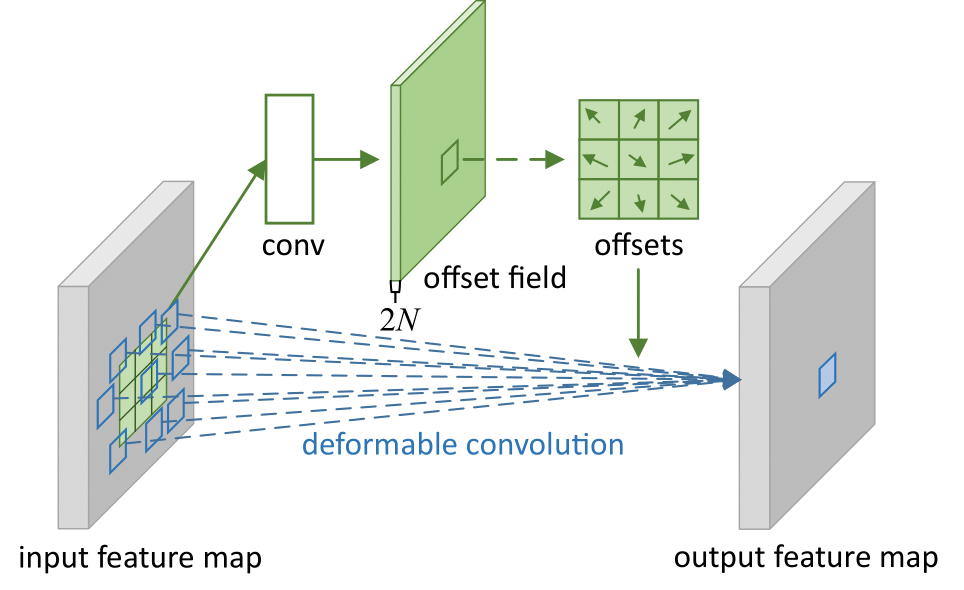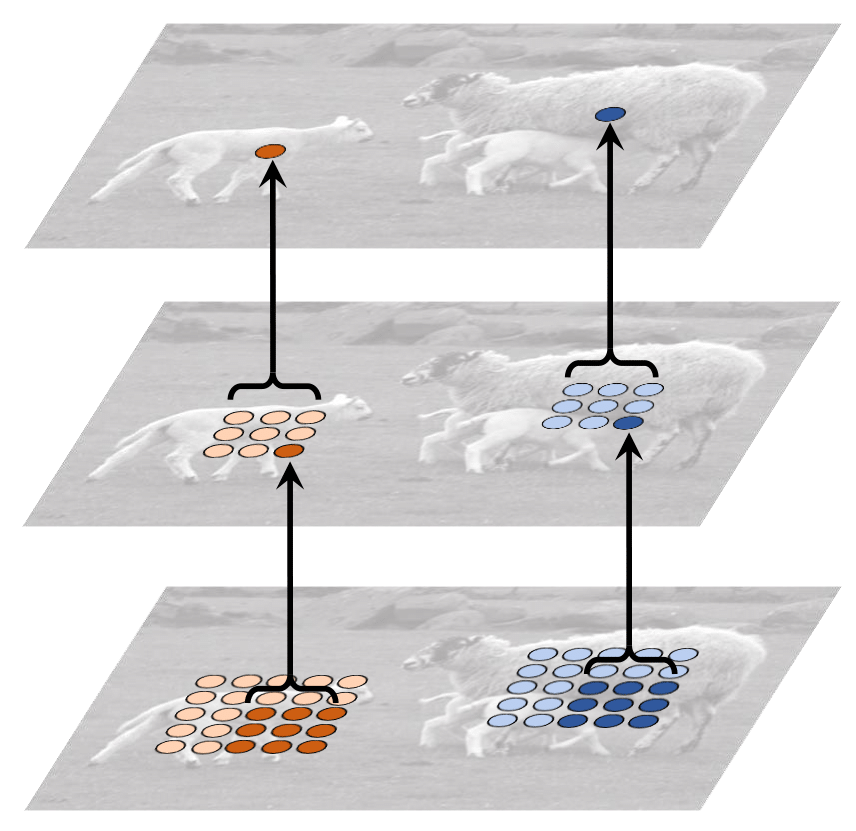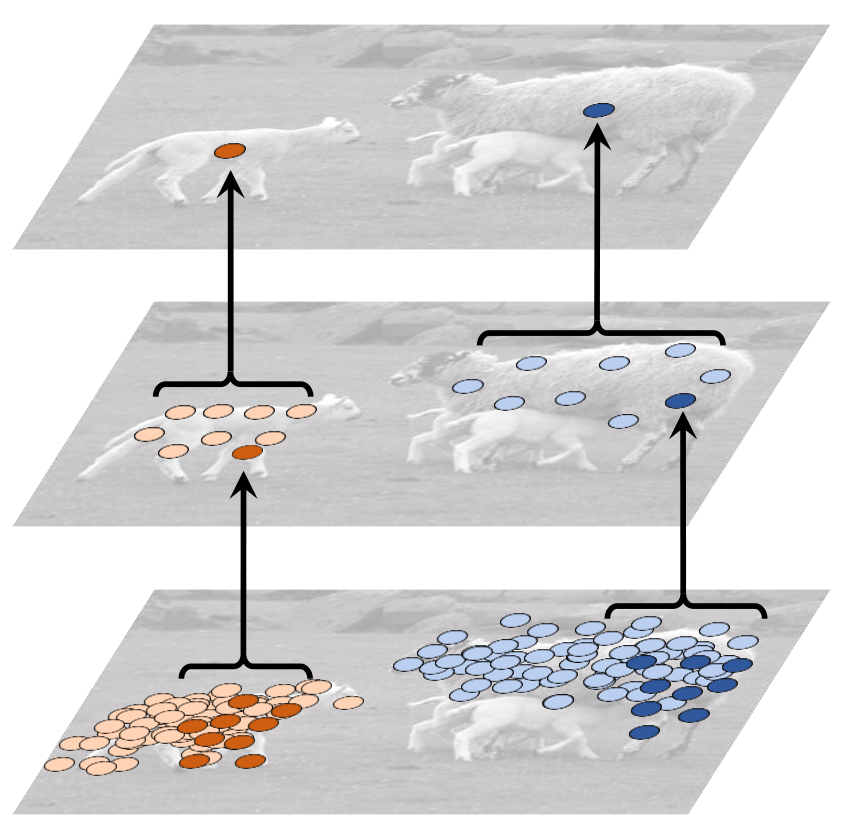# Deformable Convolutional Networks

## Deformable Convolution

Standard convolution has fixed sampling location and receptive field. To solve this problem, Deformable convolution use learnable offset.

### 2D Convolution

The standard 2D convolution consists of two steps: 1) sampling using a regular grid $\mathcal{R}$ over the input feature map $\mathbf{x}$; 2) summation of sampled values weighted by $\mathbf{w}$.

The receptive field size and dilation define the grid $\mathcal{R}$. For example, when 3 X 3 kernel with dilation 1 the grid is:

$\mathcal{R}=\{(-1, -1), (-1, 0), \ldots, (0,1), (1, 1)\}$

For each location $\mathbf{p}_0$ on the output feature map $\mathbf{y}$, 2D convolution can be denoted as followings:

$\mathbf{y}(\mathbf{p}_0)=\sum_{\mathbf{p}_n\in\mathcal{R}}\mathbf{w}(\mathbf{p}_n)\cdot \mathbf{x}(\mathbf{p}_0+\mathbf{p}_n),$

### 2D Deformable ConvolutionIn deformable convolution, the regular grid $\mathcal{R}$ is augmented with offsets $\{\Delta \mathbf{p}_n \lvert n=1,...,N\}$, where $N= \lvert \mathcal{R} \lvert$. In other words, offsets can be different per grid offset. The offsets are obtained by applying a convolution layer over same input feature map, which means the offsets are learned.

$\mathbf{y}(\mathbf{p}_0)=\sum_{\mathbf{p}_n\in\mathcal{R}}\mathbf{w}(\mathbf{p}_n)\cdot \mathbf{x}(\mathbf{p}_0+\mathbf{p}_n+\Delta \mathbf{p}_n).$

Now, the sampling is on the irregular because the offset $\Delta \mathbf{p}_n$ is typically fractional. So $\mathbf{x}(\mathbf{p}_0+\mathbf{p}_n+\Delta \mathbf{p}_n)$ is implemented via bilinear interpolation as

$\mathbf{x}(\mathbf{p})=\sum_\mathbf{q} G(\mathbf{q},\mathbf{p})\cdot \mathbf{x}(\mathbf{q}),$

where $\mathbf{p}=\mathbf{p}_0+\mathbf{p}_n+\Delta \mathbf{p}_n$ and $\mathbf{q}$ is integral positions within 2 X 2 square which is centered with $\mathbf{p}$. $G(\cdot,\cdot)$ is the bilinear interpolation kernel and can be denoted as follows:

$G(\mathbf{q},\mathbf{p})=(1- \lvert q_x-p_x \lvert ) \cdot (1- \lvert q_y-p_y \lvert )$

## Result

As Deformable convolution has offset on its grid, It can have more flexible receptive field.

Standard Convolution Deformable Convolution